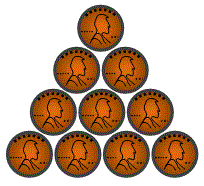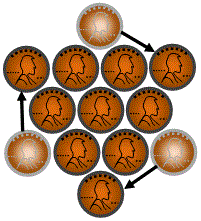## Arrow Up to Down Triangle Puzzle

 Ten coins are arranged on a flat surface so that form a triangle pointing up (as shown in the diagram). By moving only three coins to different locations on the flat surface, change the triangle so that it points down.Can you solve this puzzle? Give it a try, you can find hint by scrolling down to page.

 Hint: Notice that the coin in the center and the six coins surrounding it form a hexagon that is symmetrical in six different directions. The three coins you want to move are the three corners of the triangle.

If you still not able to solve this puzzle then scroll down and find the answer.

 Solution: Move the three coins at the corners of the triangle (as shown in the illustration), and the triangle will point down.